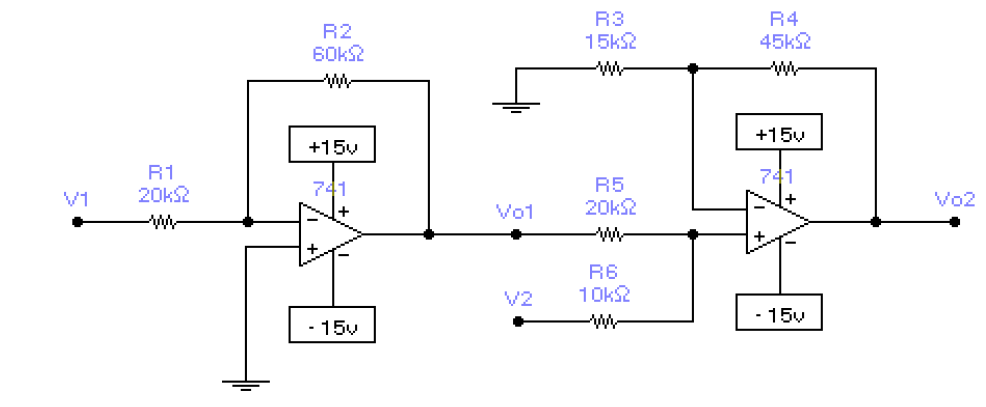###Author Topic: Simple op amp circuit need solving  (Read 676 times)

0 Members and 1 Guest are viewing this topic.

####ZeTeX

• Frequent Contributor
••• Posts: 598
• Country:• When in doubt, add more flux.##### Simple op amp circuit need solving
« on: October 18, 2017, 06:10:23 pm »
I need to find the equation for Vo2.
The answer is Vo2: -4*V1+2.666*V2
For Vo1 its easy it is just -3*V1, but for Vo2 there are 2 resistors going to the non inverting input and it is confusing, I'm not exactly sure how to solve such thing.####Kire Pûdsje

• Regular Contributor
•• Posts: 65
• Country:##### Re: Simple op amp circuit need solving
« Reply #1 on: October 18, 2017, 06:17:25 pm »
Just think of it being a voltage divider.

The following users thanked this post: ZeTeX

####ZeTeX

• Frequent Contributor
••• Posts: 598
• Country:• When in doubt, add more flux.##### Re: Simple op amp circuit need solving
« Reply #2 on: October 18, 2017, 06:42:23 pm »
Just think of it being a voltage divider.
and then what?

####Kire Pûdsje

• Regular Contributor
•• Posts: 65
• Country:##### Re: Simple op amp circuit need solving
« Reply #3 on: October 18, 2017, 06:49:40 pm »
use superposition:
for v1: -3x amp in series with voltage divider in series with 4x amp
for v2: voltage divider (reversed ratio) in series with 4x amp

Edit:
It will be clearer if you forget about the last opamp. this is just a x4 amplifier.
You already figured out vo1, and v2 is a given. Therefore what will be the voltage at the positive input?
« Last Edit: October 18, 2017, 07:00:15 pm by Kire Pûdsje »

The following users thanked this post: ZeTeX

####ZeTeX

• Frequent Contributor
••• Posts: 598
• Country:• When in doubt, add more flux.##### Re: Simple op amp circuit need solving
« Reply #4 on: October 18, 2017, 06:54:51 pm »
use superposition:
for v1: -3x amp in series with voltage divider in series with 4x amp
for v2: voltage divider (reversed ratio) in series with 4x amp
Thank you very much!

that's exactly what I did what but I tough it it was coincidence that I got the answer right.

Basically I grounded Vo1 and V2 and calculated the ration, then I wrote the eq. Vo2 = gain (Av = 4 ) * ratio
I got 2 equations that I just summed them up.
Thanks!

####w2aew##### Re: Simple op amp circuit need solving
« Reply #5 on: October 18, 2017, 06:55:24 pm »
I have two videos that may help you.  The first helps you to intuitively understand most op amp circuits, and the second is a tutorial on the "summing amp" configuration (which is what your second stage is):

Basics of Op Amp circuits:

Tutorial on the Summing Amp:
======================================